Premium

# 4.MD.A.3 Worksheets, Workbooks, Lesson Plans, and Games

#### CCSS.maths.CONTENT.4.MD.A.3

:
"Apply the area and perimeter formulas for rectangles in real world and mathematical problems."

These worksheets can help students practise this Common Core State Standards skill.

## Worksheets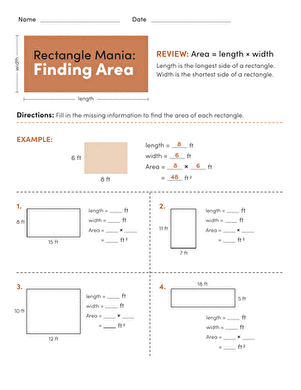Rectangle Mania: Finding Area
Worksheet
Rectangle Mania: Finding Area
In this year 5 geometry worksheet, your child will practise finding the area of a rectangle by multiplying length x width.
Year 5
Maths
Worksheet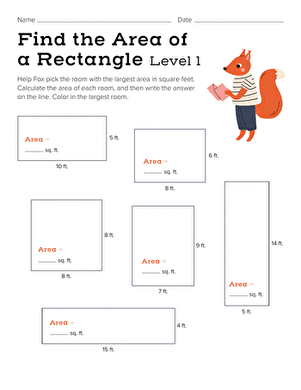Find the Area of a Rectangle: Easy
Worksheet
Find the Area of a Rectangle: Easy
Piggy needs to find a big room to house his teddy bear collection. Can your child help him figure out which room is the largest?
Year 5
Maths
Worksheet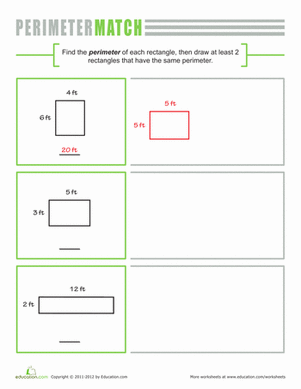Perimeter of a Rectangle
Worksheet
Perimeter of a Rectangle
Have your fourth grader stretch his maths knowledge with a geometry worksheet. He'll calculate the perimeter of a rectangle, then draw two similar shapes.
Year 5
Maths
Worksheet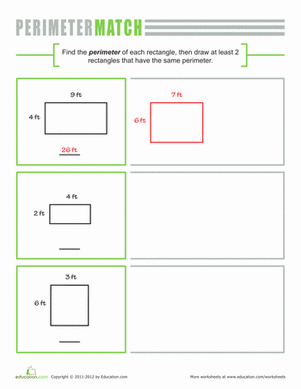Perimeter Match
Worksheet
Perimeter Match
Year five mathematicians will need to use their geometry skills to figure out the length of the pictured rectangles' perimeters, then make their own.
Year 5
Maths
Worksheet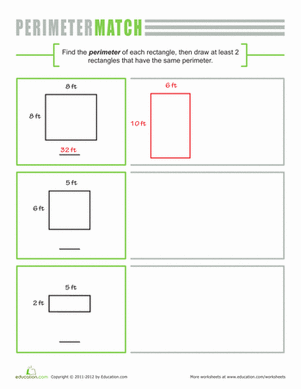Match the Perimeter
Worksheet
Match the Perimeter
Your fourth grader will need to use his geometry skills to calculate and create rectangle perimeters. He'll also improve addition and multiplication skills.
Year 5
Maths
Worksheet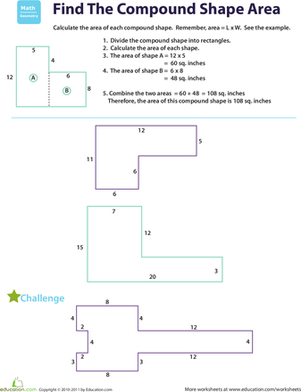Find the Area: Compound Shapes
Worksheet
Find the Area: Compound Shapes
It's not everyday that you see shapes like these! This worksheet helps your fourth grader practise finding the area of compound shapes.
Year 5
Maths
Worksheet

## Lesson Plans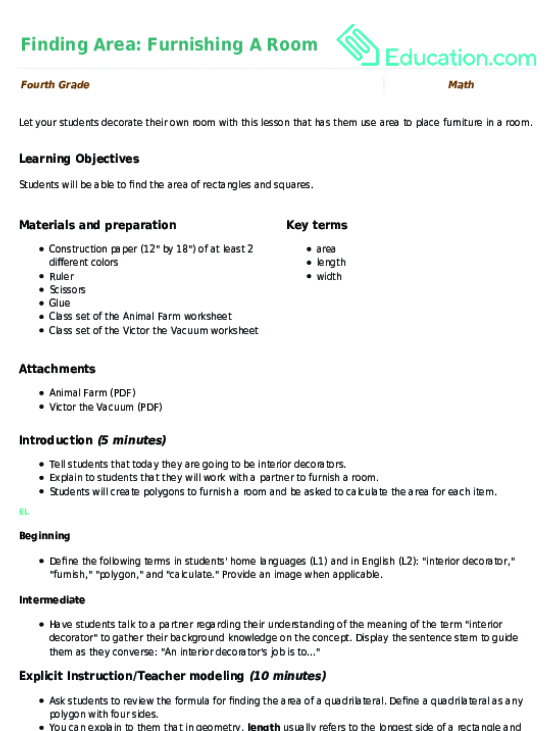Finding Area: Furnishing A Room
Lesson plan
Finding Area: Furnishing A Room
Let your students decorate their own room with this lesson that has them use area to place furniture in a room.
Year 5
Maths
Lesson planWhat's In A Name? Finding Perimeter
Lesson plan
What's In A Name? Finding Perimeter
Your students will enjoy using the perimeter formula to find the perimeter of their initials! In this lesson, they will write their initials in block letters on graph paper to include an element of art.
Year 5
Maths
Lesson plan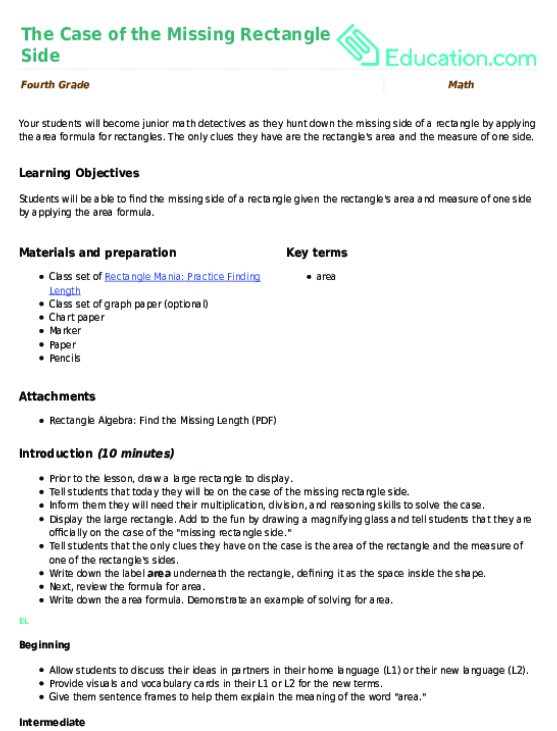The Case of the Missing Rectangle Side
Lesson plan
The Case of the Missing Rectangle Side
Your students will become junior maths detectives as they hunt down the missing side of a rectangle by applying the area formula for rectangles. The only clues they have are the rectangle's area and the measure of one side.
Year 5
Maths
Lesson plan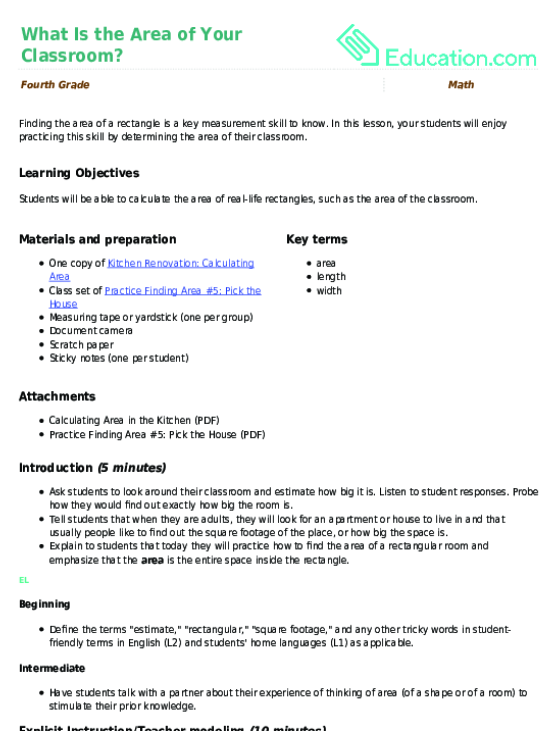What Is the Area of Your Classroom?
Lesson plan
What Is the Area of Your Classroom?
Finding the area of a rectangle is a key measurement skill to know. In this lesson, your students will enjoy practising this skill by determining the area of their classroom.
Year 5
Maths
Lesson plan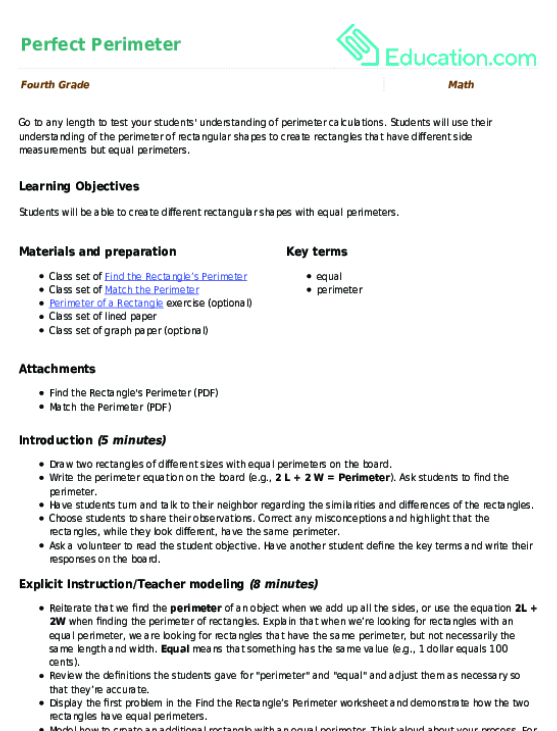Perfect Perimeter
Lesson plan
Perfect Perimeter
Go to any length to test your students' understanding of perimeter calculations. Students will use their understanding of the perimeter of rectangular shapes to create rectangles that have different side measurements but equal perimeters.
Year 5
Maths
Lesson plan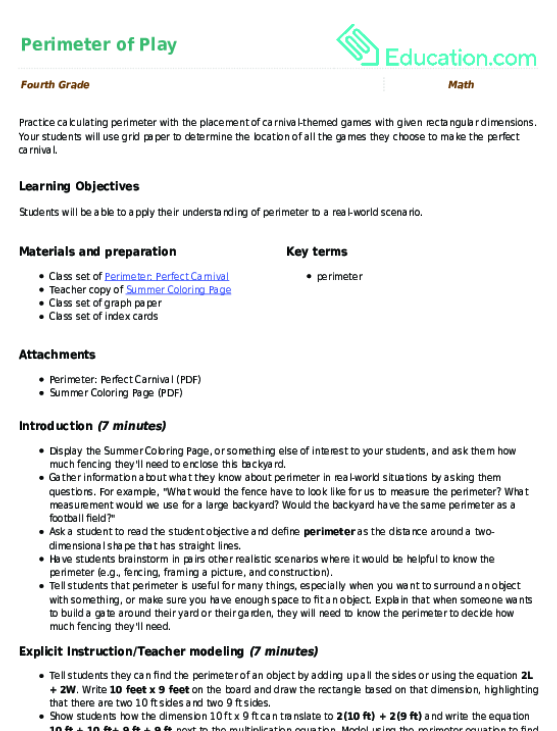Perimeter of Play
Lesson plan
Perimeter of Play
Practise calculating perimeter with the placement of carnival-themed games with given rectangular dimensions. Your students will use grid paper to determine the location of all the games they choose to make the perfect carnival.
Year 5
Maths
Lesson plan

## Workbooks

No workbooks found for this common core node.

## Games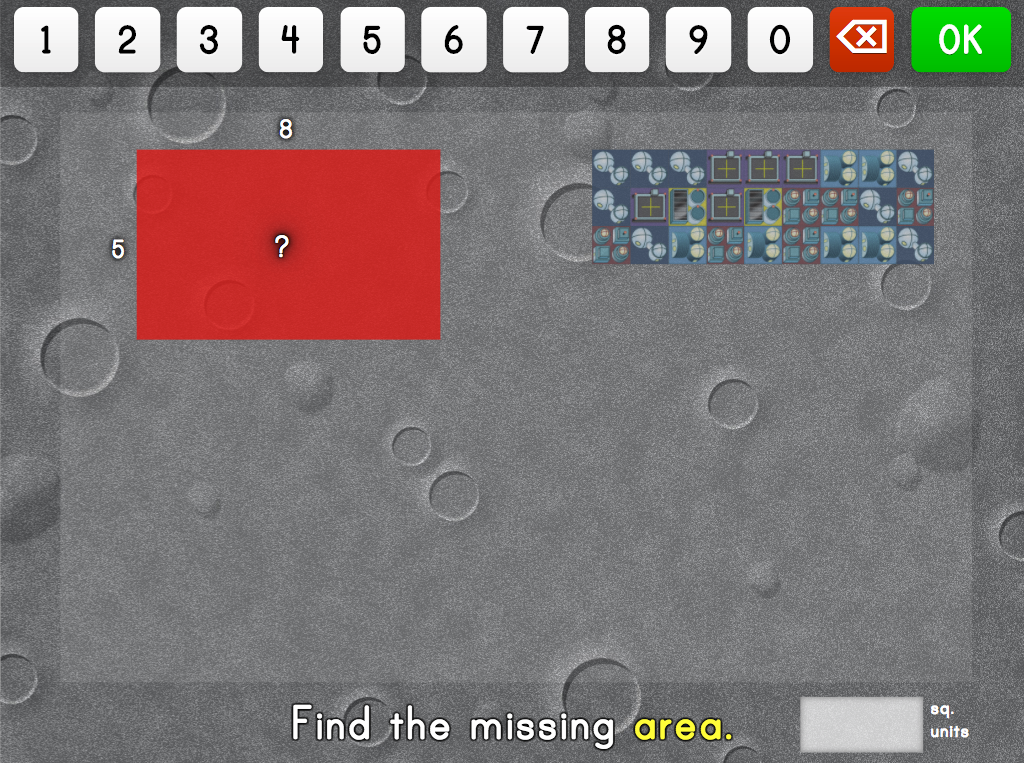City Building: Missing Area Factor
Game
City Building: Missing Area Factor
Kids practise determining area and missing lengths and widths with these space-themed visual models.
Year 5
Maths
Game

## Exercises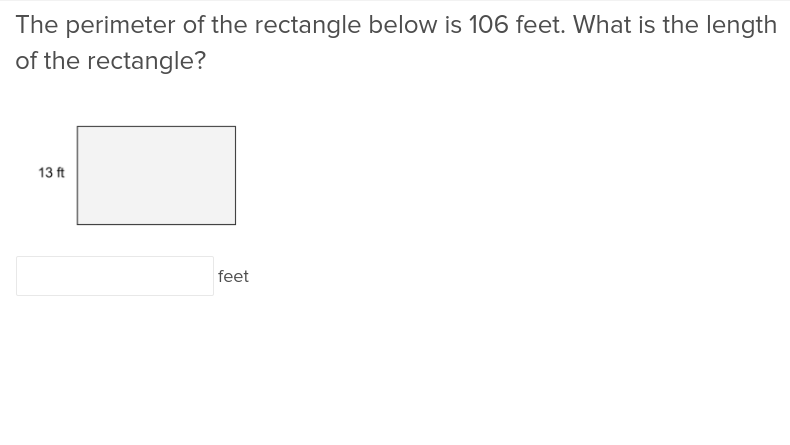Perimeter of a Rectangle
Exercise
Perimeter of a Rectangle
Students will get the bigger picture after they run through this exercise on calculating the perimeter of a rectangle.
Year 5
Maths
Exercise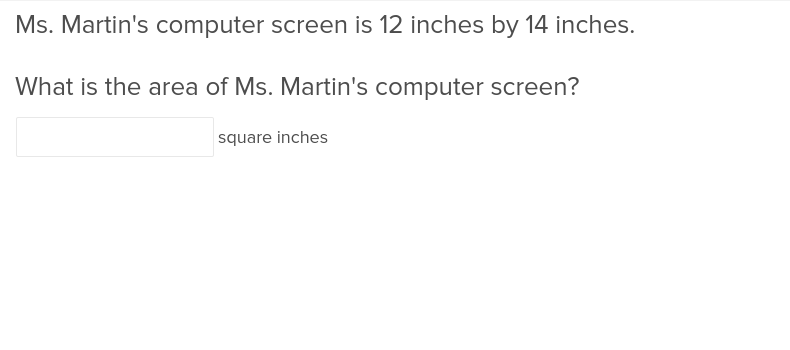Area of a Rectangle
Exercise
Area of a Rectangle
This exercise will simplify the process by which students calculate the area of a rectangle.
Year 5
Maths
Exercise

### Add to collection

Create new collection

0

### New Collection>

0Items

What could we do to improve Education.com?

Please note: Use the Contact Us link at the bottom of our website for account-specific questions or issues.

What would make you love Education.com?